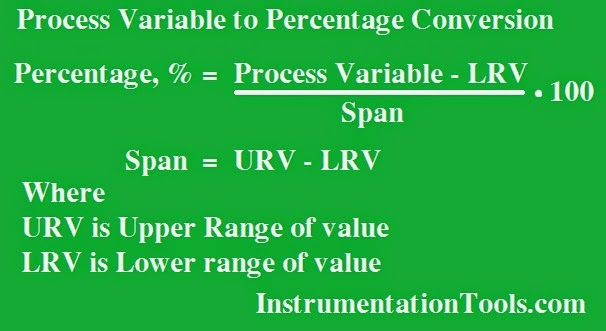# Formula for Process Variable to Percentage Conversion

Calculate the equivalent Percentage for any given Process variable. For conversion, we need transmitter Lower Range Value and Upper Range Value.

## Process Variable to Percentage ConversionFormula:

### Percentage %  =   ((Process Variable – LRV)/Span)(100)

Span = URV – LRV

Where

URV upper range value
LRV lower range value

Example:

#### An electronic Pressure transmitter is ranged -1 to 5 Bar and has a 4-20 ma. Calculate the Percentage output by this transmitter if the measured Pressure is 1 Bar

Span = 5 – (-1) = 6

Percentage % = ((Process variable – LRV)/Span)(100)

Percentage % =((1-(-1))/6)(100)

Percentage % = 33.33 %

#### Articles You May Like :

Instrumentation documents

Instrumentation Calibration Mistakes

Transmitter Span and Zero

Pressure Transmitter Applications

Formulas to calculate ma from PV

Be the first to get exclusive content straight to your email.
We promise not to spam you. You can unsubscribe at any time.

### 6 thoughts on “Formula for Process Variable to Percentage Conversion”

1.Thanks for sharing the formulas related to instrumentation.

2.Thanks for the information.

3.Thanks allot Sir

4.If conversion from MA to differential pressure any formula?????

5.6.